UDF to Extract only Number

UDF to Extract only Number from a Cell Using VBA in Microsoft Excel

In this article, we will learn how to use VBA code to extract number only from a cell with the user defined function.

Question): I need a macro to retrieve number only from a cell that contains alpha numeric data.

Following is the snapshot of the data in which column A contains alphanumeric data & expected numbers are in column B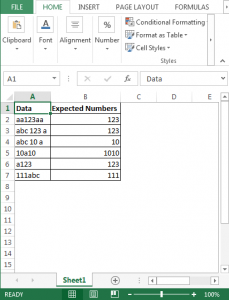In order to get only the numbers from column A; we need to follow the below steps to launch VB editor

• Click on Developer tab
• From Code group, select Visual Basic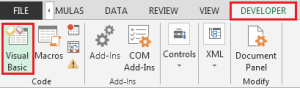Copy the below code in the Standard module

Function NumericOnly(mystr As Variant)

Dim myOutput As String, i As Integer

For i = 1 To Len(mystr)

If IsNumeric(Mid(mystr, i, 1)) Then _

myOutput = myOutput & Mid(mystr, i, 1)

Next

NumericOnly = myOutput * 1

End Function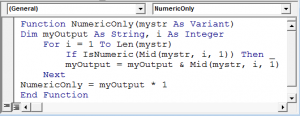• To get the output in cell B2, the formula is
• =NumericOnly(A2)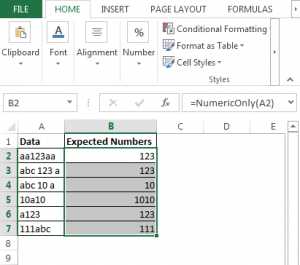In this way, we can retrieve numbers only from a cell that contains text & numbers, using VBA code.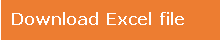Download – UDF to Extract Only Number From A Cell Using VBA – xlsm

Terms and Conditions of use

The applications/code on this site are distributed as is and without warranties or liability. In no event shall the owner of the copyrights, or the authors of the applications/code be liable for any loss of profit, any problems or any damage resulting from the use or evaluation of the applications/code.